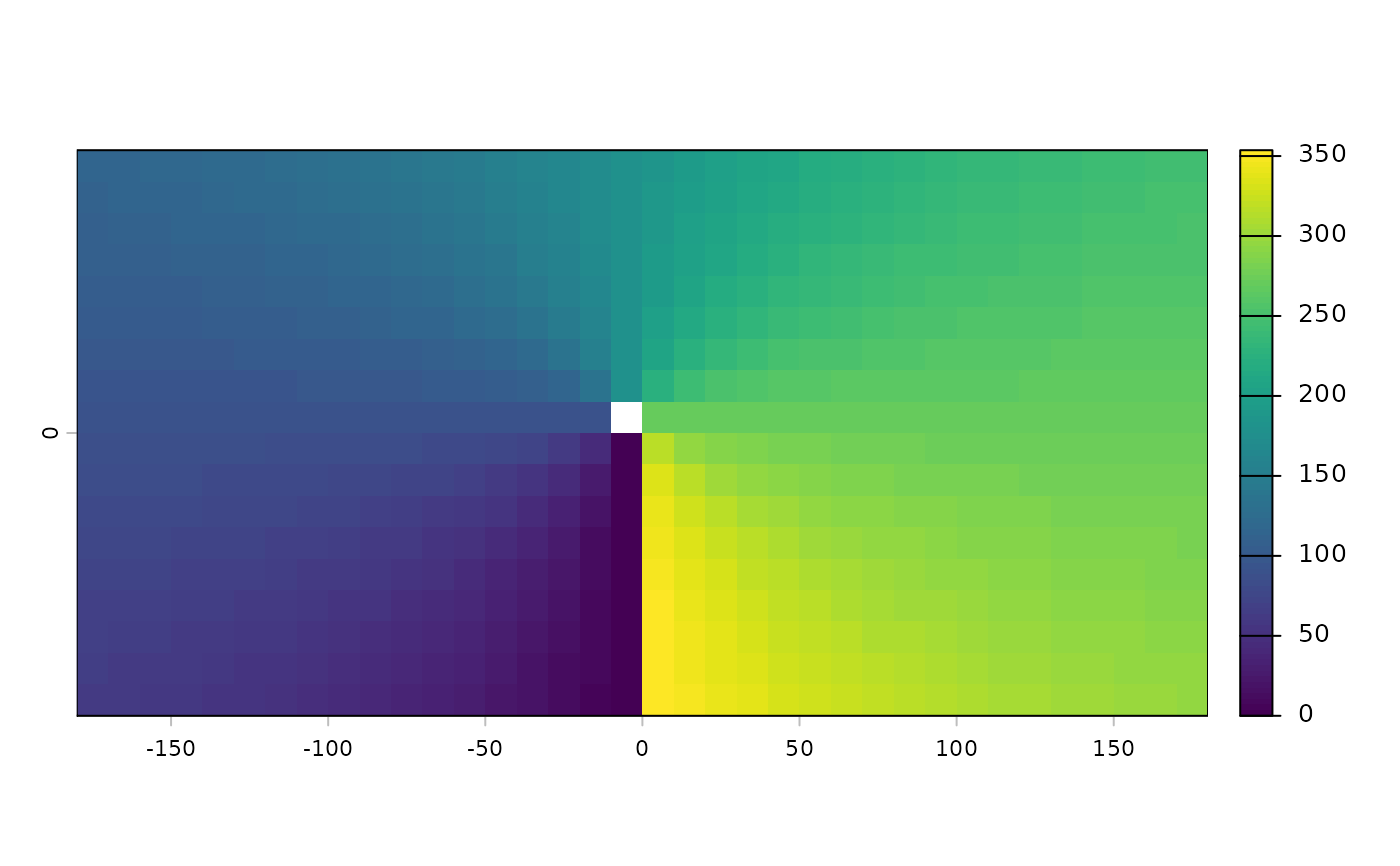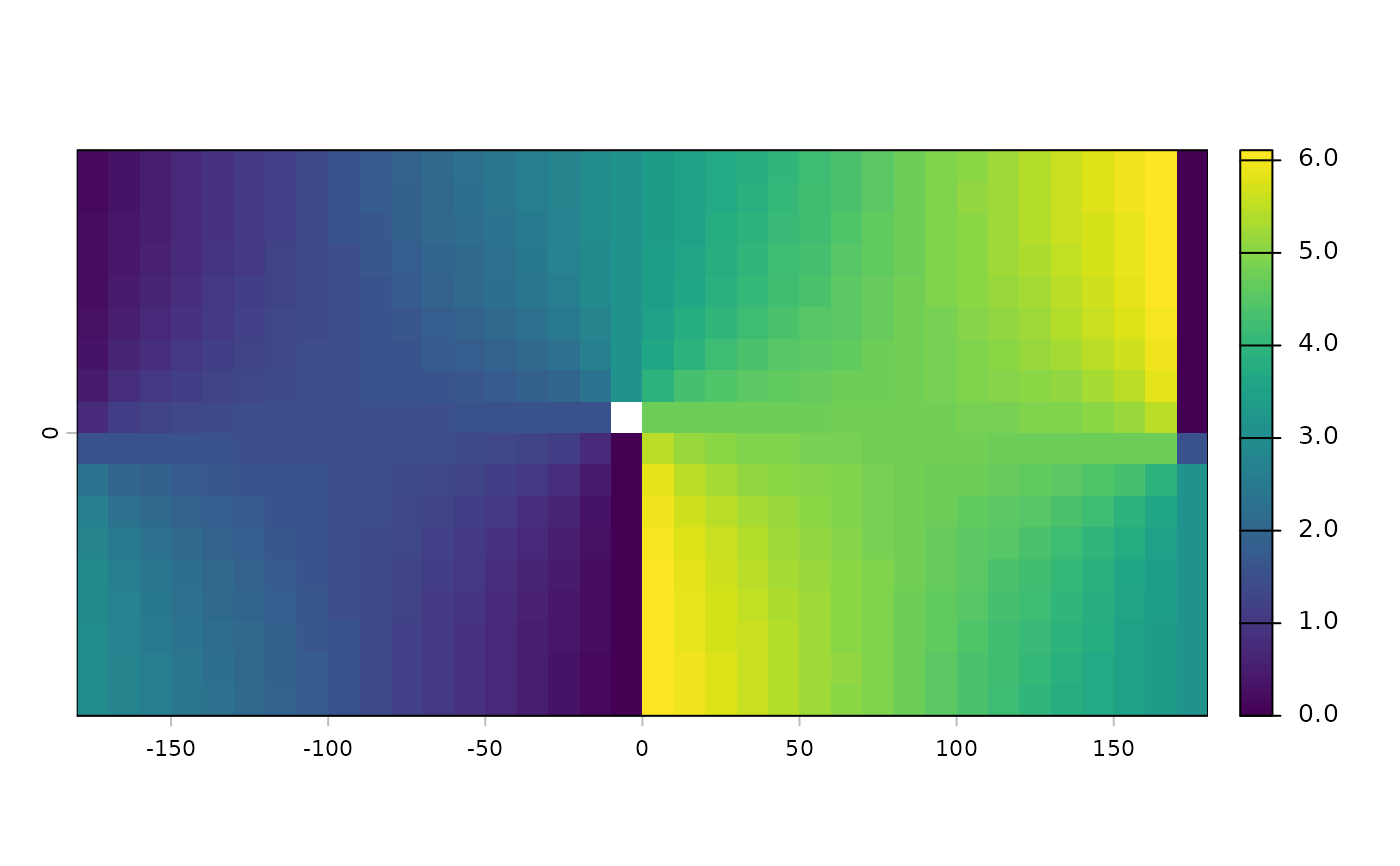The direction (azimuth) to or from the nearest cell that is not NA. The direction is expressed in radians, unless you use argument degrees=TRUE.

# S4 method for SpatRaster
direction(x, from=FALSE, degrees=FALSE, filename="", ...)

## Arguments

x

SpatRaster

filename

Character. Output filename (optional)

degrees

Logical. If FALSE (the default) the unit of direction is radians.

from

Logical. Default is FALSE. If TRUE, the direction from (instead of to) the nearest cell that is not NA is returned

...

Additional arguments as for writeRaster

## Value

SpatRaster

distance

## Examples

r <- rast(ncol=36,nrow=18, crs="+proj=merc")
values(r) <- NA
r <- 1
b <- direction(r, degrees=TRUE)
plot(b)crs(r) <- "+proj=longlat"
b <- direction(r)
plot(b)